### 2.2.1 Basic Constraints

This intuitive way, presented above, of capturing partial information about an assignment can be formally presented as a logical system of Basic Constraints. Its abstract syntax is given by: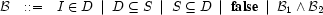It is equipped with the following inference rules.

Weakening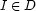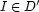where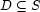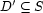where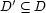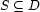whereStrengthening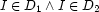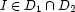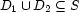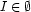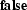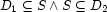whereOf course, after saturation with the above rules, for each variable there is always a most specific approximation of its assignment. For an integer variable, this is the smallest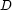such that. For a set variablethere is a largest lower boundand a smallest upper bound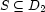. In practice, these most specific basic constraints are the only ones that the system needs to represent (all others can be derived by weakening).

Constraint Store

A constraint programming system contains a constraint store which is the (saturated) set of basic constraints representing the partial information currently known about the assignment.Determined Variables

We say that a variable is determined when its assignment has been decided. For an integer variablethis happens when its domainbecomes a singleton. For a set variable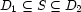this happens when its lower bound becomes equal to its upper bound.

Denys Duchier
Version 1.2.0 (20010221)